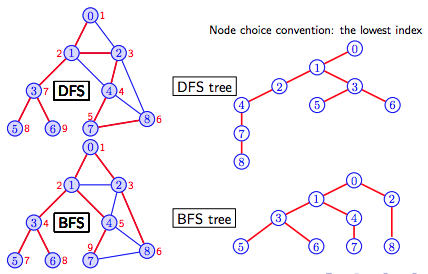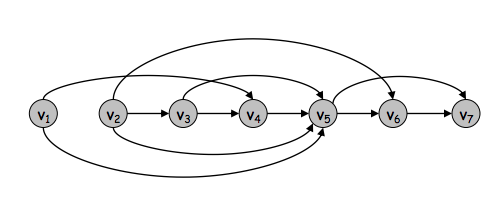### DAG数据结构与算法

#### 深度优先&广度优先 （Breadth-First Vs. Depth-first Search）#### DAGs and Topological Ordering• The reachability relation in a DAG forms a partial order, and any finite partial order may be represented by a DAG using reachability.

• The reachability relationship in any directed acyclic graph can be formalized as a partial order ≤ on the vertices of the DAG. In this partial order, two vertices u and v are ordered as u ≤ v exactly when there exists a directed path from u to v in the DAG; that is, when v is reachable from u. However, different DAGs may give rise to the same reachability relation and the same partial order. For example, the DAG with two edges a → b and b → c has the same reachability relation as the graph with three edges a → b, b → c, and a → c. Both of these DAGS produce the same partial order, in which the vertices are ordered as a ≤ b ≤ c.

• Every directed acyclic graph has a topological ordering, an ordering of the vertices such that the starting endpoint of every edge occurs earlier in the ordering than the ending endpoint of the edge. The existence of such an ordering can be used to characterize DAGs: a directed graph is a DAG if and only if it has a topological ordering. In general, this ordering is not unique; a DAG has a unique topological ordering if and only if it has a directed path containing all the vertices, in which case the ordering is the same as the order in which the vertices appear in the path.

• The family of topological orderings of a DAG is the same as the family of linear extensions of the reachability relation for the DAG, so any two graphs representing the same partial order have the same set of topological orders.

Steven S Skiena. The Algorithm Design Manual

Three important facts about topological sorting are

1. Only DAGs can be topologically sorted, since any directed cycle provides an inherent contradiction to a linear order of tasks.
2. Every DAG can be topologically sorted, so there must always be at least one schedule for any reasonable precedence constraints among jobs.
3. DAGs can often be topologically sorted in many different ways, especially when there are few constraints. Consider n unconstrained jobs. Any of the n! permutations of the jobs constitutes a valid topological ordering.
• https://en.wikipedia.org/wiki/Directed_acyclic_graph
• http://www3.cs.stonybrook.edu/~algorith/files/topological-sorting.shtml
• https://github.com/jgrapht/jgrapht/blob/master/jgrapht-core/src/main/java/org/jgrapht/traverse/TopologicalOrderIterator.java
• https://github.com/jgrapht/jgrapht/wiki/DependencyDemo
• Graphs and Digraphs BFS Priority search DAG Connectivity
• https://homes.cs.washington.edu/~jrl/421slides/lec5.pdf